• Matlab中怎么给大小未知的矩阵添加一行或者一列相同元素？ 要想解决这个问题，首先要了解两个函数 size() size函数用来获取矩阵的大小，假如有未知大小的矩阵A [m,n] = size(A);可以通过返回的m知道矩阵A的行数，...
Matlab中怎么给大小未知的矩阵添加一行或者一列相同元素？
要想解决这个问题，首先要了解两个函数
size()
size函数用来获取矩阵的大小，假如有未知大小的矩阵A
[m,n] = size(A);可以通过返回的m知道矩阵A的行数，通过n知道矩阵A的列数。
ones()
ones(M,N)可以创造行数为M，列数为N，所有元素都为1的矩阵
解决上述问题
程序如下：
A = [1,2,3;
4,5,6];

% 获取矩阵A的行数m和列数n
[m,n] = size(A)
% 创建要增加的一列元素
% 得到新矩阵

执行结果：展开全文• 话题：用MATLAB矩阵每两列求和问题详情：个3*6的矩阵a=[1 2 3 4 5 6 现要将1,2列求和,3,4列求和,5回答：a=[1:6; 1 2 3;2 4 6 1]; sum=[]; for i=1:2:5 sum=[sum a(:,i)+a(:,i+1) ]; end sum sum = 3 11 15 1...
公告： 为响应国家净网行动，部分内容已经删除，感谢读者理解。话题：用MATLAB对矩阵每两列求和问题详情：一个3*6的矩阵a=[1 2 3 4 5 6 现要将1,2列求和,3,4列求和,5回答：a=[1:6;   1 2 3;2 4 6   1];  sum=[];  for i=1:2:5 sum=[sum a(:,i)+a(:,i+1) ]; end  sum sum = 3  11 15 10 5 6 13 10 a = 1 2 3 4 5 6    1 2 3 2 4 6   1话题：matlab中如何将一个矩阵中的正负数分开,并分别求和??回答：matlab是矩阵实验室 这点和其他语言有所别 所以要充分利用矩阵运算 循环效率很低 x=randn(6); %定义6阶正态分布矩阵 y=find(x0); %找出x中大于0的元素的位置 a=x(y); %把大于的元素赋值给a s1=sum(a) %求出所有大于的元素之和 z=find(x0); %找出x中小于0的元素的位置 b=x(z); %把大于的元素赋值给b s2=sum(b) %求出所有小于的元素之和 s=s1+s2 %x的所有元素之和 s3=sum(sum(x)) %求出所有元素之和 最后两步是用于验证正确话题：矩阵元素求和的Matlab语句怎么写?举例见内回答：矩阵元素求和的Matlab语句怎么写?举例见内 sum函数  x=[2 3 4] x = 2 3 4  sum(x) ans =  Sum函数用法: B = sum(A) 返回数组A不同维数的总和。 如果A是一个向量,sum(A) 返回所有元素的总和。 如果A是一个矩阵,sum(A) 把A的列作为向量,返回一个包含每一列所有元素的总和的行向量。 如果A是一个多维数组,sum(A) 把没一维看做一个数组,返回一个行向量数组。 B = sum(A,dim) book.iLoveMatlab 沿着A的每一维计算总和用指定标量dim,dim是一个从1到N 的整数值,其中N是A的维数。 dim为1就是计算A的每一列的总和,2计算A的每一行的总和,以此类推。 B = sum(, 'double')和B = sum(, dim,'double') 在双精度话题：在matlab中高阶矩阵每行分别求和问题详情：现有15维矩阵A,想用sum(A,2)对矩阵每行求和。但发现超出范围回答：sum(A')就可以了。。求出来就是行向量的和。。。求和之后还是个向量。。 sum函数是把矩阵看成列向量来求和的。。 貌似你这样的写法也是对的啊。。。。结果是一样的。。只不过表示的一个是行向量。。一个是列向量话题：矩阵自身列项求和在MATLAB中怎样实现问题详情：怎样使列项求和后的每一项构成新的矩阵?回答：直接用sum(),就可以对矩阵的列求和,出来的是一个行向量,对应的是每列的和!参考回答：不明白你说的干什么,新的矩阵是什么维数的?话题：matlab矩阵问题详情：spl(e,g)是16X26的矩阵,我想每行对g求和,应该使用什么语句?回答：spl(1,:)表示矩阵的第一行,spl(2,:)表示矩阵的第二行,依次类推sum(spl(1,:))求和参考回答：sum(spl,2)话题：matlab矩阵元素移动求和问题详情：A=1:11; m1=length(A);n1=3; for i=1:m1-n+1 for j=n1-1; c(i)=s回答：你想要求什么和?第二个for循环都没有在循环,还有最后的plot,x,y都没有,是画不了图的。话题：在MATLAB中编写一个MEX,完成将两个矩阵的求和运算回答：(1) 矩阵加减运算 假定有两个矩阵A和B,则可以由A+B和A-B实现矩阵的加减运算。运算则是:若A和B矩阵的维数相同,则可以执行矩阵的加减运算,A和B矩阵的相应元素相加减。如果A与B的维数不相同,则MATLAB将给出错误,提示两个矩阵的维数不匹配。话题：matlab 如何对矩阵中的部分数进行求和?并在约束条件下选出问题详情：matlab 如何对矩阵中的部分数进行求和?并在约束条件下选出来?回答：小于一个数如 p=50?那随便选一个就满足条件了。话题：matlab中由循环运算得到的多个结果(矩阵),如何求和回答：能具体点吗?参考回答：如果你要生成A B C三个维数相同的矩阵,每次循环可以在每个矩阵的相同坐标(i,j)产生对应想要的结果,接着可以用D的(i,j)位置
展开全文• 1.Matlab矩阵及其操作生成两三列矩阵zeros是生成0矩阵的函数eye是生成单位矩阵冒号表达式初位是0，末位是5，步长是1(步长是1也可以省略不写)矩阵的相加减矩阵的乘除需第矩阵的行数等于第二个矩阵的列数提取...1.Matlab的矩阵及其操作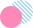生成两行三列矩阵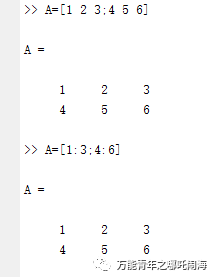zeros是生成0矩阵的函数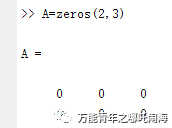eye是生成单位矩阵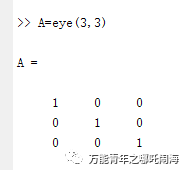冒号表达式初位是0，末位是5，步长是1(步长是1也可以省略不写)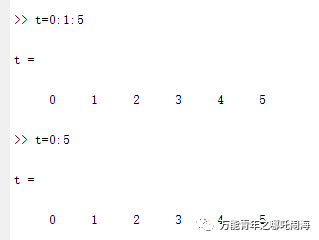矩阵的相加减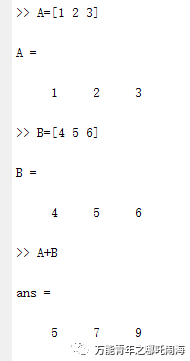矩阵的乘除需第一个矩阵的行数等于第二个矩阵的列数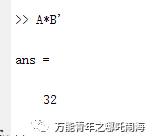提取对角线元素的函数diag()函数矩阵A的1，5为主对角线和主对角线平行往上方走的2，6叫第一条对角线4为负第一条对角线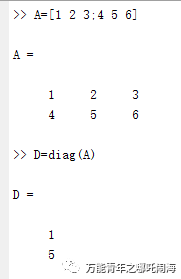提取第一条对角线和负第一条对角线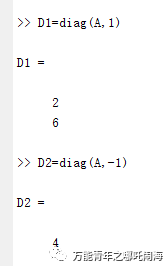利用diag函数构建对角矩阵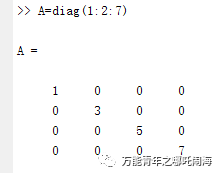对角矩阵×另一个矩阵(他就是1x矩阵A的第一行2×矩阵A第二行类似)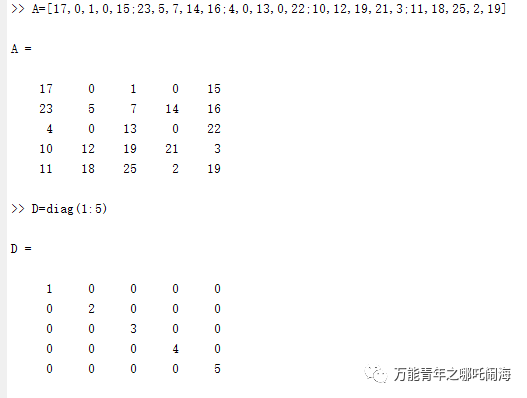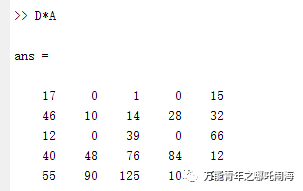上三角矩阵triu函数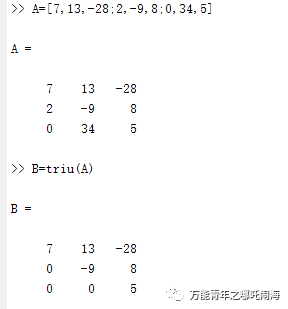选择对角线的上三角矩阵下图2代表第二条对角线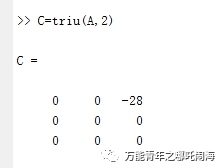矩阵的转置，就是加‘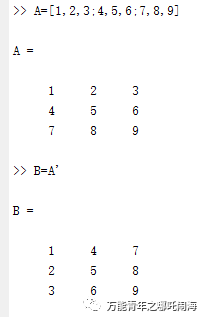矩阵的旋转90°--rot(90)把矩阵按逆时针进行旋转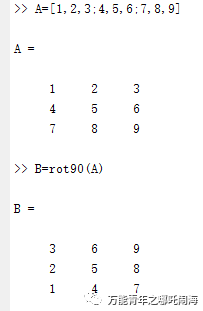4代表旋转90°4次也就是360°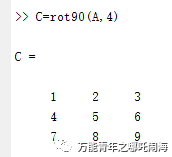矩阵的左右翻转fliplr第一列和第三列交换位置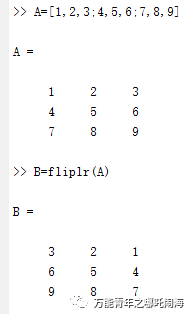矩阵的上下翻转flipud第一行和第三行交换位置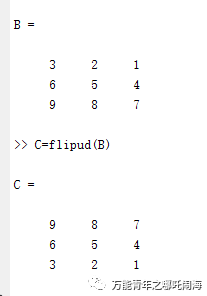矩阵的特征参数求法行列式det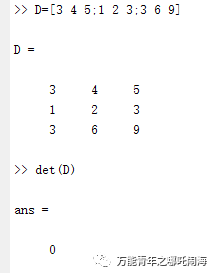矩阵分析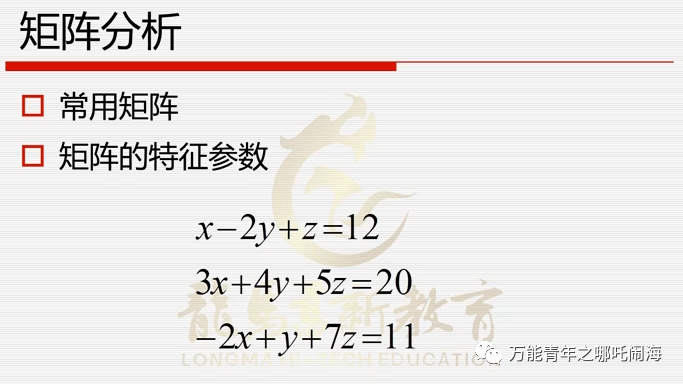左边的系数写成矩阵A，右边的常数写成矩阵b然后把A和b合成一个增广矩阵C矩阵A和矩阵C的秩是相等的，所以方程有解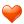矩阵的秩用rank函数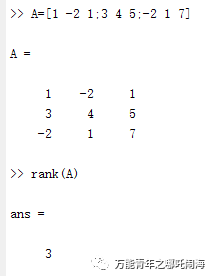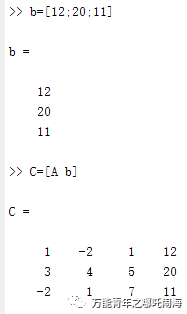求解结果(a\b  左除  ax=b      a/b  右除  xa=b)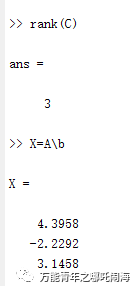求特征向量eigd是对角线为两个特征值的对角阵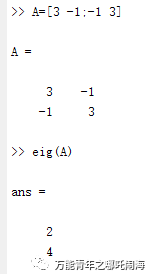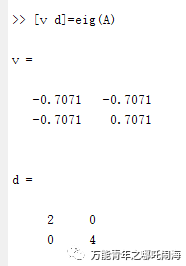矩阵的逆inv利用Matlab可以快速的求解矩阵的逆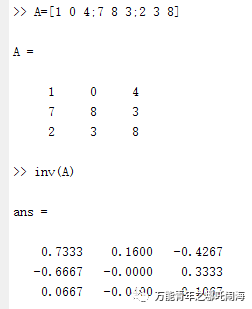把一个矩阵变成稀疏矩阵sparse以及稀疏矩阵的还原fullfull可以将稀疏矩阵转化成满的矩阵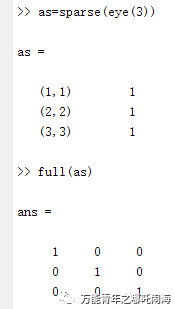sparse函数的特殊用法前两个向量代表了非零元素的行和列的下标值分别是12345而最后生成稀疏矩阵的行和列的维数是4×4的这个5表示生成稀疏矩阵最大的非零元素的个数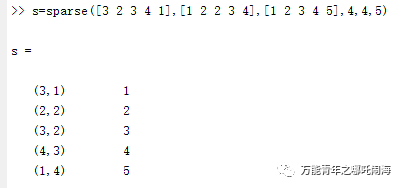nnz函数可以求出矩阵中非零元素的个数(满矩阵和稀疏矩阵都可以)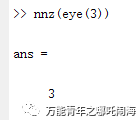spy函数可以生成一幅图图中可以显示出矩阵非零元素的分布情况(可以清楚地标出矩阵中非0元素的位置)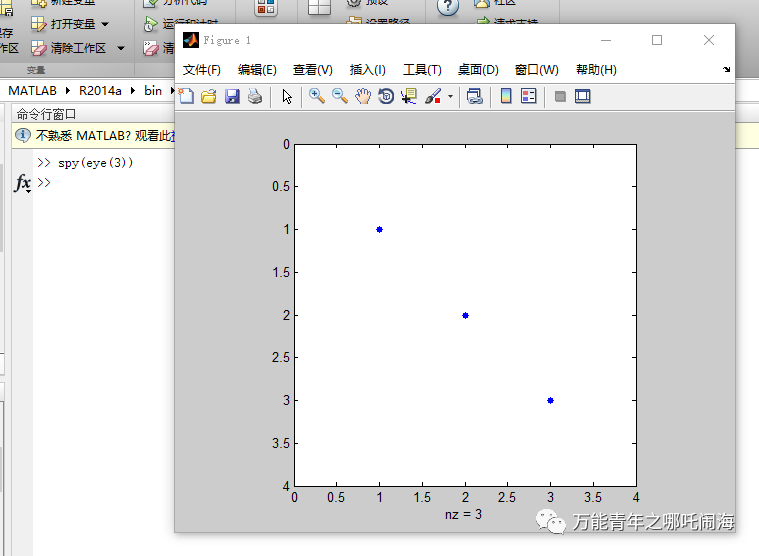对角矩阵的生成函数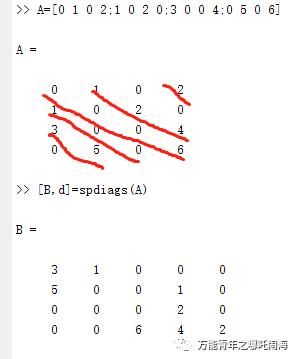生成了一个新的矩阵BB是A的每个对角线元素占一列所有非0值对角线的位置包含在向量d中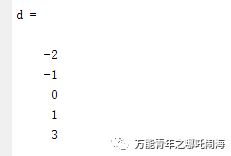2.Matlab内存管理检查内存命令(以下方法来自“度娘”搜索整理)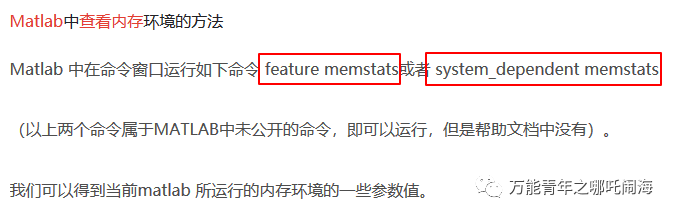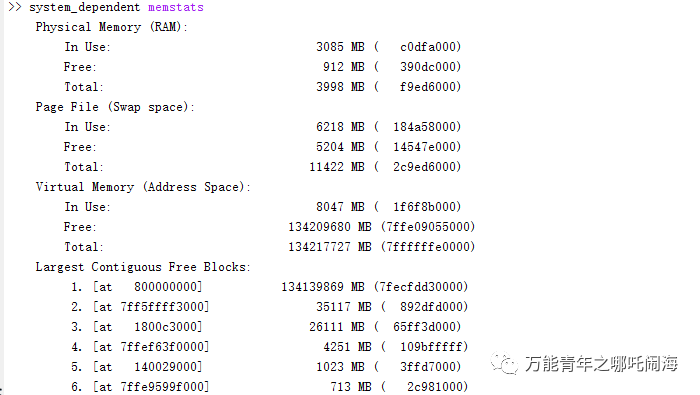当内存过多，出现“Out of Memory”问题的解决3.笔者后言个人本着学习的态度，同时也是一个不断学习的过程，以推文的形式保存也有助于自己对学习的理解和把控，也方便了其他想学习分析软体的朋友获取信息。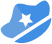扫码关注我微信：万能青年之哪吒闹海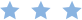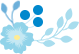END

展开全文展开全部其实这种e68a84e8a2ad62616964757a686964616f31333337396231不好用矩阵表示，因为数据格式不一样。最好的方法是matlab中的table或cell，推荐table。第一列是券商，第二列是市盈率，第三列是排名。为了实现你说的排名方法，应该在加两列，倒腾数据用，然后删除。假设券商m个，temp = cell(m,4);tab = cell2table(temp,'VariableNames',{'trader','earning','ranking','dummy1'，'dummy2'});tab.trader = {'券商1';  '券商2’}；  % 你把你提取出来的名字写成列向量。tab.earning = 市盈率列向量；tab.dummy1 = (1:m)'  % 其实是券商的序号sortrows(tab,'earning','descend') ;  %按市盈率从高到低排名，你的是从低到高，那就把descend删掉。tab.dummy2 = (1:m)' ； %排名for ii = 1:mtab.ranking{ii}=strcat(num2str(tab.dummy2(ii)),'/',num2str(m));end如果是按排名顺序，现在删掉多余的列即可；tab(:,'dummy1')=[];tab(:,'dummy2') = [];如果按券商开始的顺序排名，那么还要倒腾一下。sortrows(tab, 'dummy1');然后删除多余的列。其实更简单的是在excel中做，导入到matlab
展开全文• 矩阵的基础知识 close all; clear all; clc; %% 改变矩阵尺寸 a=eye(3);...%添加第四列，第二元素为3，其余为0 ...%将矩阵变为向量，以列为顺序，end表示最后个元素 %% 改变矩阵形状 a=magi
• >> A=[1,2,3] A = 1 2 3 >> A=[A;4 5 6] //插入 A = 1 2 3 4 5 6 >> A=[A 7;8] 错误使用 horzcat 要串联的数组的维度不一致。 >> c=[7;8] c = 7 ...
• 2*3矩阵，a(1,[1 3]) 取第一行的 第一列和第三列数据 a=[1,2,3;4,5,6] a = 1 2 3 4 5 6 b= a(1,[1 3]) b = 1 3 循环拼接 单个添加 c=[] >> c(end+1,1) = a(1,1) c = 1 >> c(end+1,1) ...
• 5.2 移动数据参考视频: 5 - 2 - Moving Data Around (16 min).mkv...怎样把数据存入矩阵？如何对矩阵进行相乘？如何保存计算结果？如何移动这些数据并用数据进行操作？进入我的 Octave 窗口，我键入，得到我们之...
• 实验步骤 1.了解MATLAB工作窗口各部分的作用 （1）命令行窗口 可以在此输入各种指令、函数和表达式等。 （2）工作区 ...4.编写脚本、调用脚本、脚本中添加注释 （见代码部分） 5.矩阵数据的二划分 a＝m
• Matlab提取矩阵规模信息（行数及列数） （1）s=size(A), 当只有一个输出参数时，返回一个向量，该行向量的第一个元素时矩阵的行数，第...（3）size(A,n)如果在size函数的输入参数中再添加一项n，并用1或2为n赋值，...
• MATLAB中自带的高斯白噪声的两个函数 MATLAB中产生高斯白噪声非常方便，可以直接应用两个函数...y = wgn(m,n,p) 产生个mn列的高斯白噪声的矩阵，p以dBW为单位指定输出噪声的强度。 y = wgn(m,n,p,imp) ...
• 如4成3的矩阵与7乘3的矩阵，竖直连接，因为每一行都有三列。 扩展矩阵大小的两种方法： Ø 连接新的元素到当前矩阵 Ø 将数据存储到当前矩阵范围之外 注意：如果打算不断的扩展矩阵...扩展 struct 存储
• MATLAB中自带的高斯白噪声的两个函数 MATLAB中产生高斯白噪声非常方便，可以直接应用两个函数...y = wgn(m,n,p) 产生个mn列的高斯白噪声的矩阵，p以dBW为单位指定输出噪声的强度。 y = wgn(m,n,p,imp) ...
• %最后一行添加一个元素。。 s=[]; for i=1:6 if i>2 s=[s;i]; end end s %最后一行添加一整行 s1=[]; A=reshape([1:16],4,4); for i=1:4 s1=[s1;A(i,:)]; end s1 %添加一列，该列只有一个元素。 s3=[]; for...
• MATLAB中产生高斯白噪声的两个函数MATLAB中产生...1. WGN：产生高斯白噪声y = wgn(m,n,p) 产生个mn列的高斯白噪声的矩阵，p以dBW为单位指定输出噪声的强度。y = wgn(m,n,p,imp) 以欧姆(Ohm)为单位指定负载阻抗...
• MATLAB中自带的高斯白噪声的两个函数MATLAB中产生...1. WGN：产生高斯白噪声y = wgn(m,n,p) 产生个mn列的高斯白噪声的矩阵，p以dBW为单位指定输出噪声的强度。y = wgn(m,n,p,imp) 以欧姆(Ohm)为单位指定负...输出信噪比公式
• 目前想实现在矩阵添加一行的功能，如 A=[1 2 3; 4 5 6] B=[1 2 3] A=[A;B] 在eigen中可以使用repmat定义一个新的临时矩阵，然后将A,B放进去 但是我想要像matlab一样简单，百度不到，google了一下 ...
• ## Matlab

2015-07-21 16:46:00
Ctrl + R 加上层注释（行首添加"%空格"）Ctrl + T 去掉层注释（行首去掉...zeros(M，N) 表示的是M*N列的0矩阵 ones()——生成全1阵 MATLAB array indices start from one, not zero\ A*B does a matrixmul...
• 接着执行该文件并添加一行新的代码为“legend(‘a’,’b’);”即可。matlab中stem函数用法stem(Y) 将数据序列Y从x轴到数据值按照茎状形式画出，以圆圈终止。如果Y是一个矩阵，则将其每一列按照分隔方...
• size（）：获取矩阵的行数和列数（1）s=size(A),当只有一个输出参数时，返回一个向量，该行向量的第一个元素时矩阵的...（3）size(A,n)如果在size函数的输入参数中再添加一项n，并用1或2为n赋值，则 size将返回...size
• find函数用来获取向量或矩阵中非0元素的索引...看个例子：A是向量，B是个列向量，将B中非0元素的索引添加到A中 A = [1 2 3]; B = [0;0;0;4;5;6]; A = [A, find(B)]; 结果出现错误： Error using horz...
• 寻找个矢量的哪些元素在另外个矢量中出现过，也可以找矩阵的哪些（列）在另矩阵中出现过 2. setdiff 寻找在a中却不在b中的元素（矢量），或列（矩阵） 3. figure('numbertitle',
• timetable 数据类型：使用常规时间步长存储时间时节省内存 7、应用构建 应用设计工具：在应用设计工具画布上添加和配置日期选择组件 uigridlayout 函数：使用网格布局管理器配置应用布局 滚动容器：针对图、面板、...
• 1、size（）：获取矩阵的行数和列数 （1）s=size(A), 当只有一个输出参数时，返回一个向量，该行向量的第一个元素时矩阵的行数...（3）size(A,n)如果在size函数的输入参数中再添加一项n，并用1或2为n赋值，则 siz...
• size（）：获取矩阵的行数和列数 1、s=size(A),当只有一个输出参数时，返回一个向量，该行向量的第一个元素是矩阵的行数，...3、size(A,n)如果在size函数的输入参数中再添加一项n，并用1或2为n赋值，则 size将返回...# matlab矩阵添加一行matlab 订阅